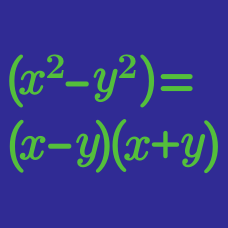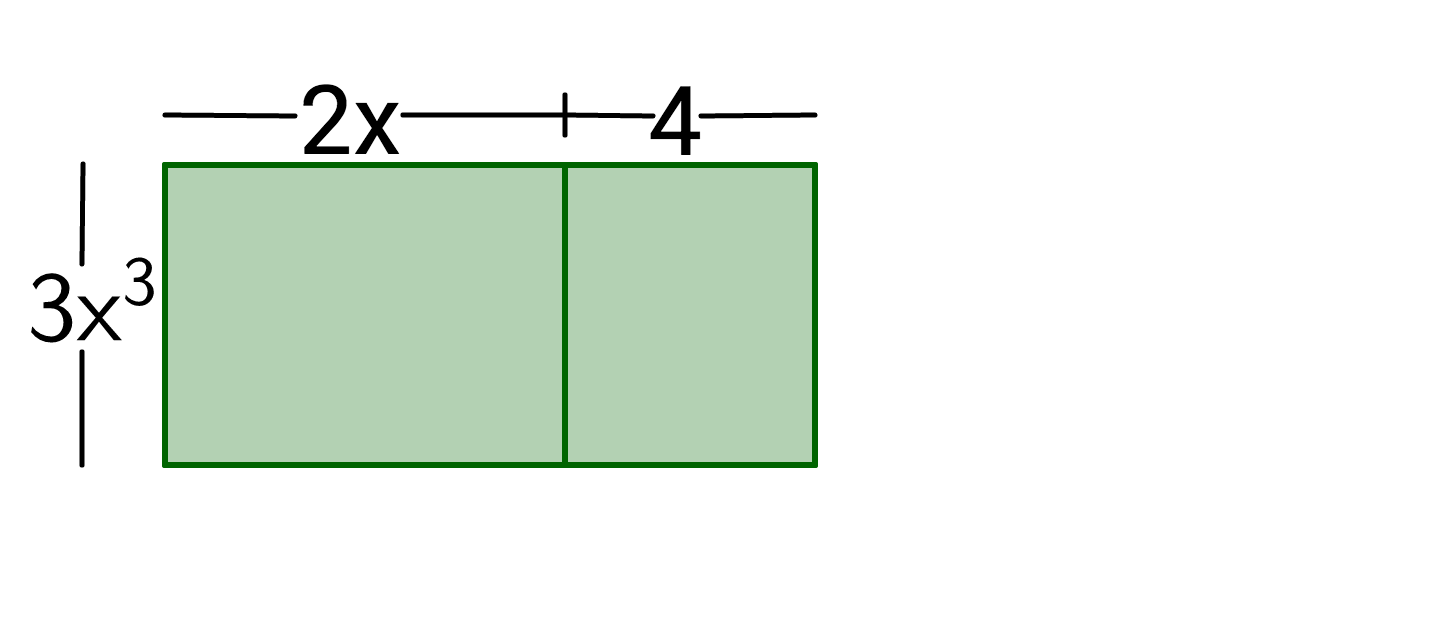Algebra

# Factoring Polynomials Warmup

True or False?

$x(x + 1) + x(x + 2) = 2x^2 + 3x$

Are these expressions equivalent?

$(2x^2 - x) + (8x - 4)$

and

$x(2x - 1) + 4(2x - 1)$

$x^4 - y^6 = \, ?$

Is $(x + 2)$ a factor of $(x^3 - 8)?$

(HINT: If $(x - 4)$ is a factor of an expression, then 4 is a solution to the equation created when that expression equals 0.)If the dimensions of the figure are located along the rectangular figure's perimeter, what is the figure's area?

×